Konovalov E. O., Chernitska O. V., Atanova M. J.

Oles Honchar Dnipropetrovsk National University

APPLICATION OF KORNIYCHUK 'S INEQUALITY TO THE UPPER BOUND DIMENSIONAL LINEAR WIDTHS

SUBCLASS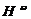OF SPACES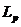(p = 4)

Inequality which is valid for all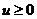for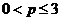was established and proven by M.P Korniychuk [1, p. 225-226]: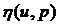=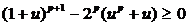. (1)

Inequality is examined for significance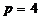. The continuous function f(t),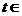[а,b] is considered and has the view such as on the drawing (Fig. 1).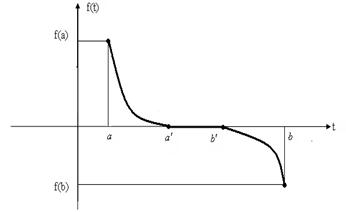Fig. 1. Function f(t)

Function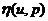becomes negative in the intervals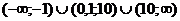. If you want to do the inequality (1) correct, you will require compliance next equality: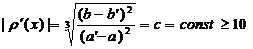.   (2)

So, if function f(t) such as on the drawing, for p=4 and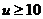, next inequality will correct: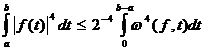, (3)

where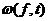– is the modulus of continuity of function f(t).

The list of references:

1. Korneychuk N. P. Splines in theory of approximations. – M.: Science, Home edition physical and mathematical literature, 1984. – 352 p.